Function Repository Resource:

# BinaryIteratedLog

Get the binary iterated logarithm of a positive number

Contributed by: Wolfram|Alpha Math Team
 ResourceFunction["BinaryIteratedLog"][z] gives the binary iterated logarithm of z.

## Details

The binary iterated logarithm is also known as inverse binary tetration. It is defined to be the smallest (integer) number of times that logarithm base 2 must be applied to a number to yield a result less than 1.

## Examples

### Basic Examples

BinaryIteratedLog is the inverse of binary tetration (repeated exponentiation):

 In:=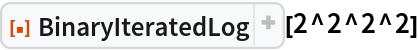Out=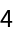A slightly larger input shows a step-like jump in the value of BinaryIteratedLog:

 In:=Out=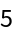Make a table of the binary iterated logarithm of the first 30 integers:

 In:=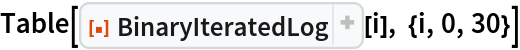Out=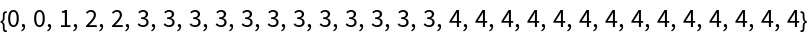### Properties & Relations

BinaryIteratedLog gives zero for values less than 2:

 In:=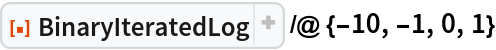Out=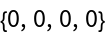## Publisher

Wolfram|Alpha Math Team

## Version History

• 4.0.0 – 23 March 2023
• 3.1.0 – 11 May 2021
• 3.0.0 – 24 January 2020
• 2.0.0 – 06 September 2019
• 1.0.0 – 10 July 2019